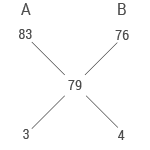# Sample Aptitude Questions of Infogain

DIRECTIONS for questions 1 – 4: Solve the equations given below and answer
if X < Y
if X > Y
if X  ≥ Y
if X ≤  Y
or no relation can be established
1. 6X2 + 31X + 35 = 0
2Y2 + 3Y + 1 = 0

6X2 + 31X + 35 = 0
Factorize 6X2 + 21X +10X +35=0
So we get factors of X = -7/2 and -5/3
2Y2 + 3Y + 1 = 0
After factorize we get
Y = -1 and -1/2
So conclusion D follows
2. 2X2 - (4 + 13) X + 2 13 = 0
10Y2 - (18 + 513) Y + 9 13 = 0

2X2 - (4 + √13) X + 2 √13 = 0
After factorizing we get
2X - √13X - 4 √13X+ 2 √13 = 0
X = 2 and √13/2
10Y2 - (18 + 5 √13) Y + 9 √13 = 0
Y = √13/2 and 9/2
Hence conclusion D follows
3. 2X2 + 9X + 10 = 0
4Y2 + 28Y + 45 = 0

2X2 + 9X + 10 = 0
Factorizing
2X2 + 5X +4X +10=0
We get X = -5/2 and -2
4Y2 + 28Y + 45 = 0
Factorizing we get
4Y2 + 18Y +10Y +45 = 0
Y= -9/2 and -5/2
Hence conclusion C follows
4. 15X2 - 11X - 12 = 0 20Y2 - 49Y + 30 = 0

15X2 - 11X - 12 = 0
After factorizing we get
15X2 - 20X+9X - 12 = 0
We get X=4/3 and -3/5
20Y2 - 49Y + 30 = 0
After factorizing we get
20Y2 - 25Y-24Y + 30 = 0
We get Y = 5/4 and 6/5
Hence conclusion E follows.
5. A and B are partners in a business. They invest in the ratio 5:6, at the end of 8 months A withdraws. If they receive profits in the ratio of 5 : 9, find how long B's investment was used?
1. 12 months
2. 10 months
3. 15 months
4. 14 months
5. 18 months

Ratio of profit is always distributed in the ratio of their investment and time.
5unit × 8 months:6 units × B’s months = 5:9
So B’s investment time = 12 months
6. There are 3 red balls, 4 blue balls and 5 white balls. 2 balls are chosen randomly. Find probability that 1 is red and the other is white.
1. 5/22
2. 5/23
3. 7/22
4. 4/9
5. None of These

There are 3 red balls, 4 blue balls and 5 white balls. 2 balls are chosen randomly.
probability that 1 is red and the other is white = 3/12× 5/11 = 5/44
7. According to a new plan rolled out by H1SP Bank, the rate of simple interest on a sum of money is 8% p.a. for the first two years, 10% p.a. for the next three years and 6% p.a. for the period beyond the first five years. Simple interest accrued on a sum for a period of eight years is Rs. 12,800. Find the sum.
1. Rs. 24,000
2. Rs. 16,000
3. Rs. 15,000
4. Rs. 13,500
5. None of These

Let the sum of money be x.
So interest in first two years = 8×2 = 16% of x
Interest in next three years = 10×3 =30% of x
Interest in next five years = 6×3 = 18% of x
Total interest = 64% of x = Rs 12,800
We get x = Rs 20,000
8. Three Science classes A, B and C take a Life Science test. The average score of students of class A is 83. The average score of students class B is 76. The average score of class C is 85. The average score of class A and 8 is 79 and average score of class B and C is 81. Then the average score. Of classes A, B and C is
1. 80
2. 80.5
3. 81
4. None of these9. A hemispherical bowl of internal diameter 54 cm contains a liquid. The liquid is to be filled in cylindrical bottles of radius 3 cm and height 9 cm. How many bottles are required to empty the bowl?
1. 221
2. 343
3. 81
4. 243
5. None of these

Area of a hemispherical bowl = 2/3 ×π ×r3
Area of a cylinder = πr2h
Area of a cylinder = n × Area of a hemispherical bowl
2/3 ×π ×273 = n ×π×32×9
we get n = 162
10. Solve : (638 + 9709 - 216) ÷ 26 = ?
1. 275
2. 365
3. 420
4. 300
5. 390